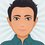# Some questions of Number Theory

I wish to know how to solve these problems. Any help will be appreciated!

## Question 1

Find the total number of natural numbers $$n$$ for which $$111$$ divides $$16^n-1$$ where $$n$$ is less than $$1000$$.

Answer: $111$

## Question 2

Find the remainder when $(1!)^2+(2!)^2+(3!)^2 \dots (100!)^2$ is divided by $1152$.

Answer: $41$

## Question 3

Find the remainder when $3^{21}+9^{21}+27^{21}+81^{21}$ is divided by $3^{20}+1$.

Answer: $60$

I have also provided the answers, but I wish to know the proper method to solve this, and if there is some trick to solve these questions in general, any opinions are welcome!Note by Vinayak Srivastava
3 months ago

This discussion board is a place to discuss our Daily Challenges and the math and science related to those challenges. Explanations are more than just a solution — they should explain the steps and thinking strategies that you used to obtain the solution. Comments should further the discussion of math and science.

When posting on Brilliant:

• Use the emojis to react to an explanation, whether you're congratulating a job well done , or just really confused .
• Ask specific questions about the challenge or the steps in somebody's explanation. Well-posed questions can add a lot to the discussion, but posting "I don't understand!" doesn't help anyone.
• Try to contribute something new to the discussion, whether it is an extension, generalization or other idea related to the challenge.

MarkdownAppears as
*italics* or _italics_ italics
**bold** or __bold__ bold
- bulleted- list
• bulleted
• list
1. numbered2. list
1. numbered
2. list
Note: you must add a full line of space before and after lists for them to show up correctly
paragraph 1paragraph 2

paragraph 1

paragraph 2

[example link](https://brilliant.org)example link
> This is a quote
This is a quote
    # I indented these lines
# 4 spaces, and now they show
# up as a code block.

print "hello world"
# I indented these lines
# 4 spaces, and now they show
# up as a code block.

print "hello world"
MathAppears as
Remember to wrap math in $$ ... $$ or $ ... $ to ensure proper formatting.
2 \times 3 $2 \times 3$
2^{34} $2^{34}$
a_{i-1} $a_{i-1}$
\frac{2}{3} $\frac{2}{3}$
\sqrt{2} $\sqrt{2}$
\sum_{i=1}^3 $\sum_{i=1}^3$
\sin \theta $\sin \theta$
\boxed{123} $\boxed{123}$

Sort by:

You might need to learn Modular arithmetic which is the basic requirement for such questions. Then explore number theory practice on brilliant.

• Modular Arithmetic
• Parity of Integers
• Number Theory
• Chinese Remainder Theorem
• Linear Diophantine Equations
• Fermat's Little Theorem
• Lucas' Theorem
• Sum of Squares Theorems
• Euler's Totient Function
• Euler's Theorem
• Finding the Last Digit of a Power
• Order of an Element
• Primitive Roots
• Lifting The Exponent

- 3 months ago

The answer to these specific questions can be found on the internet as well

- 3 months ago

Can you tell the solution of second one also? I think I understand the solution of the third problem given on Quora. Thanks for these two anyway!

- 3 months ago

Umm.. I wasn't able to find for the second one. I've seen a similar question where the expression is to be divided by 100. And that is easy because after 10!, all the numbers are divisible by 100. And you can do it that way. Do tell if you find an answer to the second question

- 3 months ago

OK, I'll wait for others to see this. Thanks for these two solutions!

- 3 months ago

I found it! I just observed that $(6!)^2$ and so on is divisible by $1152$. So I just squared the first five terms on calculator, and got the correct answer! Your comment was a good hint on what to do! Thanks a lot!

- 3 months ago

Yeah Márton has done that. Great!

- 3 months ago

I did it first :( But good thinking by you both!

- 3 months ago

I solved the problems as @Mahdi Raza linked them. The solution for the second question: $1152=2^7\cdot 3^2$. So from $(6!)^2$ each square is divisible by 1152. Therefore the solution is $(1+2^2+6^2+24^2+120^2)\;mod\;1152=41$.

Nice!

- 3 months ago